# Money Balling Cricket: Bayesian methods to get parameters

Sabermetrics has been useful in predicting baseball outcomes and has been used by professional teams, to achieve better results. The story has been well documented in the movie “Money ball”. The idea is to use statistics to find hidden value in players, and make better decisions in team building.

Now the obvious question arises is there a way to use statistics to find “hidden value” in other sports, namely cricket. The purpose of this article to explore this question and see how one can go about doing that. The article is inspired and adapted from this research paper: Modelling and simulation for one-day cricket. The paper is an interesting read for anyone interested in cricket and also statistics.

Most of the approach is directly adapted from the paper but I decided to make some slight changes in my methodology:

• The paper is written with one-day cricket in mind, my approach has been adapted for T-20
• The paper makes adjustments for the second innings — in order to reduce complexity my approach is innings agnostic. Since, the end goal for me was to analyze individual players not complete matches.

In this first part I will show you how you can generate estimates for players that can be then used for predicting outcomes and also simulating interesting scenerios.

# Data

In order to apply this approach you need ball by ball data; you need the batsman who was batting, the bowler who was bowling, the number of balls played in the innings and also the outcome of every ball. Fortunately, a dataset was available on https://cricsheet.org/. They store the data in the form of yaml files, you can pick any dataset considering you can extract yaml files into a dataframe. If you don’t know how to do that, you can download the dataset that I used from here:https://github.com/ArslanS1997/Cricket

Once prepared the data should look like this, where you at least have the over ,outcome of the ball, the batsman and the bowler.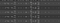My dataset after extraction

Your dataset can look slightly different but as long as it has the four elements, you should be able to go ahead.

In order to get the pass the dataset into stan (the statistical library we will be using) you will need to assign each batsman and bowler an index number and with every ball you need to assign the number for the batsman and the bowler.

# Model

The model begins with creating a discrete probability distribution over outcomes. It considers 7 outcomes for each ball. As shown below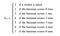Outcome of each ball

It considers 1 as the outcome of a wicket, 2 as the outcome that ball results in zero runs and 3–7 correspond to scoring 1–6 runs with 5 runs being excluded as that is very rare event and can bias the results. It is a multinomial distribution. So essentially we need to find the probability that any ball b results in the kth outcome. The probability depends on the batsman, bowler, wickets taken and the outcomes of b-1 balls played up until ball b. Which in mathematical notation is

where i is the batsman, j is the bowler, w is the wicket, b is the ball and k is the outcome. These probability estimates are essentially what we are trying to find.

Now in order to assign the outcomes in the dataframe you can create a function as shown below.

`def outcome(row):if(row['The_wicket']==False):     return 1elif(row['batsmen runs']==0):     return 2elif(row['batsmen runs']==1):     return 3elif(row['batsmen runs']==2):     return 4elif(row['batsmen runs']==3):     return 5elif(row['batsmen runs']==4):     return 6elif(row['batsmen runs']==6):     return 7else:     return np.nan#You can use pandas apply function to assign this to each rowdf['outcome'] = df.apply(outcome, axis=1)`

The paper says in order to estimate these probabilities you can use Bayesian latent variable model based on ordered logistic regression. Ordered Logistic regression is different from vanilla logistic regression that it outputs an ordinal outcome or the Prob(X≤k) rather than a simple categorization or Prob(X=k). Latent variable essentially means a variable that isn’t observed in the data but the distribution of the variable can be found using observed variables. The paper describes a variable U as the “quality of batting outcomes” and says that every ball results in an outcome k given U takes a value between certain thresholds.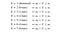Batting outcomes

# What is U?

The paper defines this latent variable in terms of four things previously mentioned the batsman, the bowler, wickets (situation) and ball currently being faced. In order to keep things simple the paper first does this simple derivation, where we only consider the batsman and the bowler.

In this you mu_i describes the ability of the batsman and mu_j describes the ability of the bowler, plus an error term. The higher the value of mu_i, mu_j the more better the player is. In order to find the probability of X≤k we follow this mathematical procedure. Where a_k is the threshold for the kth outcome and the function F() is the logistic/sigmoid function. The sigmoid function outputs a number between 0,1 , so thus can be used to create a probability distribution over all values.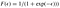The Logistic function aka Sigmoid function

This all seems very confusing especially if this is the first time you’re dealing with high level Bayesian modelling but the key takeaway you need to get from the above derivation is that when we have three things mu_i,mu_j and the threshold we can get the probability of X≤k by simply plugging these in the logistic function. And in order to compute probability X=k, we need the subtract the Prob(X≤k) with Prob(X≤k-1).

The paper then extends this concept to include situation( wickets and overs played) and introduces a set of threshold values for every situation. It divides each match into nine situations.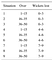Situations for One day cricket

The way to read this is that if there are 1–15 overs balled in the innings & 0–3 wickets are lost the situation of the match is 1, similarly if 36–50 overs are bowled and 7–9 wickets are lost then we are in 9. You can get the idea.

Since, our dataset is for T-20 matches we need to adjust these assignments, we only have 20 overs per innings so, I’ve adjusted the situations as shown below:

`# Index variable denotes the overs playeddef assign_situation(row):  if(row['Wickets_lost']>=0 and row['Wickets_lost'] <=3):    if(row['index']>0 and row['index']<=5):      return 1    elif(row['index']>5 and row['index']<=15):      return 2    elif(row['index']>15):      return 3  elif(row['Wickets_lost']>3 and row['Wickets_lost'] <=6):    if(row['index']>0 and row['index']<=5):      return 4    elif(row['index']>5 and row['index']<=15):      return 5    elif(row['index']>15):      return 6  elif(row['Wickets_lost']>6 and row['Wickets_lost'] <=10):    if(row['index']>0 and row['index']<=5):      return 7    elif(row['index']>5 and row['index']<=15):      return 8    elif(row['index']>15):      return 9`

Now in the adjusted model which incorporates the situation looks like this

Where the subscript l denotes the situation, and a_lk is the threshold for the lth situation and the kth outcome, delta_l is a variable that incorporates the “pressure” as the situation of match becomes more difficult.

These delta_1, delta_2 are two new parameters we need to estimate, which will give us the value of delta_l for each situation. It is highly advised that you pause at this moment to think about each point mentioned and also read the paper along with my coded post, in order to get a better understanding. Just as before in this new model, if we want to compute the Prob(X=k), we need to the following, we need to substract Prob(X≤k) and Prob(X≤k-1) or in the form of the logistic function

# Estimating Parameters

Now we know what we need to do if we have all the mu’s, a_lk, and deltas but how do we estimate them. Since, this is a bayesian setting we need to give these parameters some initial distributions. The paper uses these prior distributions for all our parameters.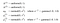priors for all parameters

In order to compute the posterior estimates from these priors you will need a statistical software package. You can use any software capable of doing so but I preferred to use stan, while the paper uses WINBUGS. Stan computes these posterior distribution by using Markov Chain Monte Carlo (MCMC) methods, a very standard way of computing bayesian parameters.

You can use stan as library in both Python and R. All you need is the Pystan and Rstan packages and also you will need to specify the priors and the relationship of the model to stan in it’s own statistical programming language, which is pretty similar to C++.

If you’re new to stan, you can just use the stan code I used to specify the model. Or if you want to learn how this works, please check out the stan documentation : https://mc-stan.org/users/documentation/

`import pystan modelcode = """data {    int<lower=1> N;     int<lower =1> BatNum;    int<lower =1> BowlNum;    int BatIndex[N];    int BowlIndex[N];    int<lower=1,upper=9> l[N];    int<lower=1,upper=7> F[N];    int<lower=1> index[N];    int matchballs;}parameters {ordered alk_tilda;    real<lower=0> sigma;    real<lower=0> tau;    real mu_1_tilda[BatNum];    real mu_2_tilda[BowlNum];               real<lower=0,upper=1> delta_1;   real<lower=0,upper=1> delta_2;}transformed parameters{real delta;real mu_1[BatNum];real mu_2[BowlNum];ordered alk;      for(n in 1:9){   delta[n] = D(l[n],delta_1,delta_2);   alk[n] = sqrt(1/sigma)*alk_tilda[n];   }for (n in 1:BatNum){mu_1[n] = sqrt(1/tau)*mu_1_tilda[n];}for (n in 1:BowlNum){mu_2[n] = sqrt(1/tau)*mu_2_tilda[n];}}model {  //    vector[N] s;    delta_1 ~uniform(0,1);    delta_2 ~uniform(0,1);          sigma ~ gamma(1,1);     tau ~ gamma(1,1);    mu_1_tilda ~ normal(0,1);    mu_2_tilda ~ normal(0,1);   for(t in 1:9){      alk_tilda[t] ~ normal(0,1);    } for(i in 1:N){  s[i] =  mu_1[BatIndex[i]] + delta[l[i]] - mu_2[BowlIndex[i]];       } for(i in 1:N){F[i]~ ordered_logistic(s[i]',alk[l[i]]); }}"""sm = pystan.StanModel(model_code=modelcode)`

The upper code allows you to compile your stan model and next you need to supply the data and run stan’s sampling. This is the code for training or running the sampler.

`# First creating a list of all the batsman and bowlersbatsman =complete_data_train['batsman'].unique()bowler = complete_data_train['bowler'].unique()#Assigning an index to each bowler and batsman respectivelybowler = [[bowler[i-1],i] for i in range(1,len(bowler)+1)]batsman = [[batsman[i-1],i] for i in range(1,len(batsman)+1)]#Situation of each balll = [int(x) for x in complete_data_train['situation']]#the actual outcomeF = [int(x) for x in complete_data_train['outcome']]#Functions that assign each batsman and bowler with their respective index in our dataframecomplete_data_train['BatIndex'] = complete_data_train.apply(assign_num, theList= batsman, string = 'batsman',axis=1)complete_data_train['BowlIndex'] = complete_data_train.apply(assign_num, args=(bowler,'bowler',),axis=1)#converting that into a listBatIndex = [int(x) for x in complete_data_train['BatIndex2']]BowlIndex = [int(x) for x in complete_data_train['BowlIndex2']]BatNum = int(len(batsman))BowlNum = len(bowler)N = int(len(complete_data_train))matchballs = 240index = [int(x) for x in complete_data_train['balls']]data = {'N':N,'l':l,'F':F,'BatIndex':BatIndex,'BowlIndex':BowlIndex,'BatNum':BatNum,'BowlNum':BowlNum,'matchballs':matchballs,'index':index}#Stan fit object will have all the trained parameters.fit = sm.sampling(data, iter=2000, chains=4,n_jobs=1,verbose=True,refresh=100,control={'max_treedepth': 10})`

In the dataset I had around 25000 balls,86 unique batsman and 76 unique bowlers. That makes the total parameters to estimate equal to 1+1 (deltas) + 9(6) (a_lk) + 86(batsman) and 76(bowlers) which equals 218 parameters to estimate. At the current iteration settings it took 3–4 hours for training the dataset.

After training is over we take the mean of stans outputs like this

`# Extract output alk=fit.extract()['alk']mu1 = fit.extract()['mu_1']mu2 = fit.extract()['mu_2']del1 = fit.extract()['delta_1']del2 = fit.extract()['delta_2']# Assign mean #using previously made lists with name and index of bowler & batsman # you can assign the mu_i and mu_jbatsman = pd.DataFrame(batsman, columns=['name','number'])bowler = pd.DataFrame(bowler, columns=['name','number'])batsman['mean'] = [x for x in mu1.mean(axis=0)]bowler['mean'] = [x for x in mu2.mean(axis=0)]# estimates for deltasd1 = del1.mean(axis=0)d2 = del2.mean(axis=0)# estimates for thresholds alk=pd.DataFrame(alk.mean(axis=0),columns=['mean_'+str(x) for x in range(1,7) ])`

The output of each of the dataframes should be like this: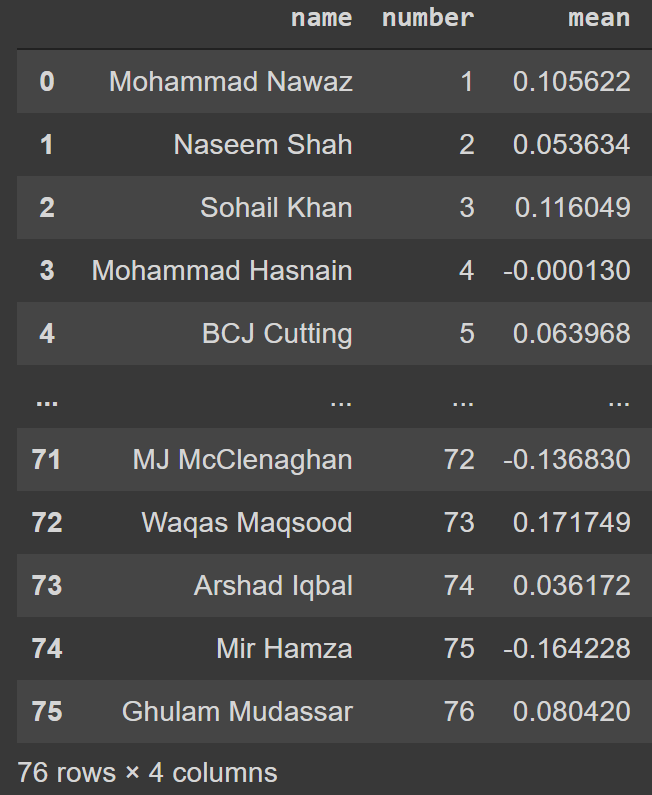Fig 1. Thresholds for all 9 situations and 7 outcomes (only need 6 values for 7 outcomes), Estimates for batsman and Estimates for bowlers

# Stay Tuned

This was certainly challenging demo, however we are just reaching the most interesting bit that is to use these estimates to predict ball by ball outcomes and also run simulations to see what will happen in interesting scenarios. Follow me so you can get notified when the next part come out. In the meantime, stay curious!

## MLearning.ai

Data Scientists must think like an artist when finding a solution

## MLearning.ai

Data Scientists must think like an artist when finding a solution, when creating a piece of code.Artists enjoy working on interesting problems, even if there is no obvious answer.

Written by

## Arslan Shahid

Interested in everything!## MLearning.ai

Data Scientists must think like an artist when finding a solution, when creating a piece of code.Artists enjoy working on interesting problems, even if there is no obvious answer.

## More From Medium

Medium is an open platform where 170 million readers come to find insightful and dynamic thinking. Here, expert and undiscovered voices alike dive into the heart of any topic and bring new ideas to the surface. Learn more

Follow the writers, publications, and topics that matter to you, and you’ll see them on your homepage and in your inbox. Explore

If you have a story to tell, knowledge to share, or a perspective to offer — welcome home. It’s easy and free to post your thinking on any topic. Write on Medium